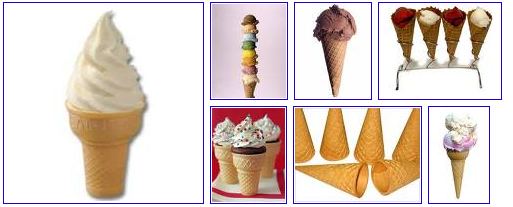Exercises on the Axiomatic Method [Hvidsten Chapter 1]
Last revised 29may13
\begin{document}
\maketitle\section{Introduction}
We here collect exercises appropriate for the introduction to axiomatic
geometry based on Hvidsten's first chapter, but expanded by lesssons in
this section of the course. All exercises on this page should be attempted
by the student, and the completed exercises entered into the Journal. A
selection of these problems will be collected for correction and advice.
Some will be tested on exams or the final. The union, intersection,
and complements of these subsets are non-empty.

This document is updated frequently and should be consulted periodically for
additions. It also contains comments and advice on doing the problems.

You are directed to justify your solution to
the problems on finite geometries (aka toy geometries) using models rather
than attempting to construct proofs directly from the Axioms. Few
beginners can do this successfully, and failed attempts are too difficult
for your grader to correct. Intead, recall from the lessons that a categorical
axiom system (one which has a unique model, up to an isomorphism)
has the additional convenient property that theorems in the axiom system can be
"read-off" the model. Thus, in solving
the problems below you should first construct a model (don't forget to justify your
discovery) and then you may assume that the axiom system modelled is categorical.

The reason for this deviation from the Hvidsten Text is this. To deduce all of the
theorem of Geometry (especially Non-Euclidean Geometry) from a set of axioms is beyhond
the scope of this course. Instead, we follow the analytic method of using models, after
accepting the fact that the Geometries we study are categorical.

\subsection{Three Point Geometry}
\textbf{ Exercises 1.4.3 through 1.4.5 } pertain to the finite incidence
geometry consisting of exactly 3 points. This subject is treated
in the lesson on \textit{ Toy Geometries }. You should translate the
axioms by replacing  "children", "likes", "flavors"
with "points", "lines" and "incidence" and prove the problems using
a model for the axioms instead of attempting a discursive argument.

\subsection{Peano's Axioms for Arithmetic}
Ever since Euclid presented the first set of axioms for geometry, mathematicians
wondered about an axiomatic treatment of arithmetic. For a long time it was
thought that the rules of arithmetic are so obvious and uncontroversial that
they are simply part of the human mind. When a similar philosophical opion
regarding geometry was proven false in the 19th century, axioms for arithmetic
became serious business. Guiseppe Peano gave these
\begin{itemize}
\item \textit{Primitives:} Numbers $\mathbb{N}$, Successor
\item \texttt{Peano 1:} $1 \in \mathbb{N}$
\item \texttt{Peano 2:} $(\forall n \in \mathbb{N})(\exists n' \in \mathbb{N})( n' \mbox { is the unique successor to } n )$
\item \texttt{Peano 3:} $(\forall n, m \in \mathbb{N})(n \ne m \implies n' \ne m')$
\item \texttt{Peano 4:} $(\forall n \in \mathbb{N})(1 \ne n')$
\item \texttt{Peano 5:}
If  $S \subset \mathbb{N}$ for which
\begin{enumerate}
\item $1 \in S$
\item $(\forall n \in \mathbb{N})(n \in S \implies n' \in S )$
\end{enumerate}
then $S = \mathbb{N}$.
\end{itemize}
As with Euclid, the interesting axiom is the Fifth Peano Axiom, better
known as the \textit{ Principle of Finite Induction.}

Hvidsten's \textbf{ exercises 1.4.3 through 1.4.12} treat Peano's axioms.

\subsection{Exercises 1.5.1 - 1.5.3}
Hvidsten's exercise sections contain questions best answered by a
thoughtful essay. These are appropriate for students taking the course
for the fourth credit hour.

\subsection{Four Point Geometry}
\textbf{Exercises 1.5.4 through 1.5.7} pertain to the finite incidence
geometry consisting of exactly 4 points. This subject is treated
in the lesson on \textit{ Toy Geometries }. You should do these
problems using a model instead of attempting to compose as
discursive argument.

\subsection{Geometric Arithmetic}
\textbf{Exercise 1.6.3} treats geometric arithmetic. This and similar
examples easily located on the WWW are particularly important for
current or future teachers of school math.

\end{document}